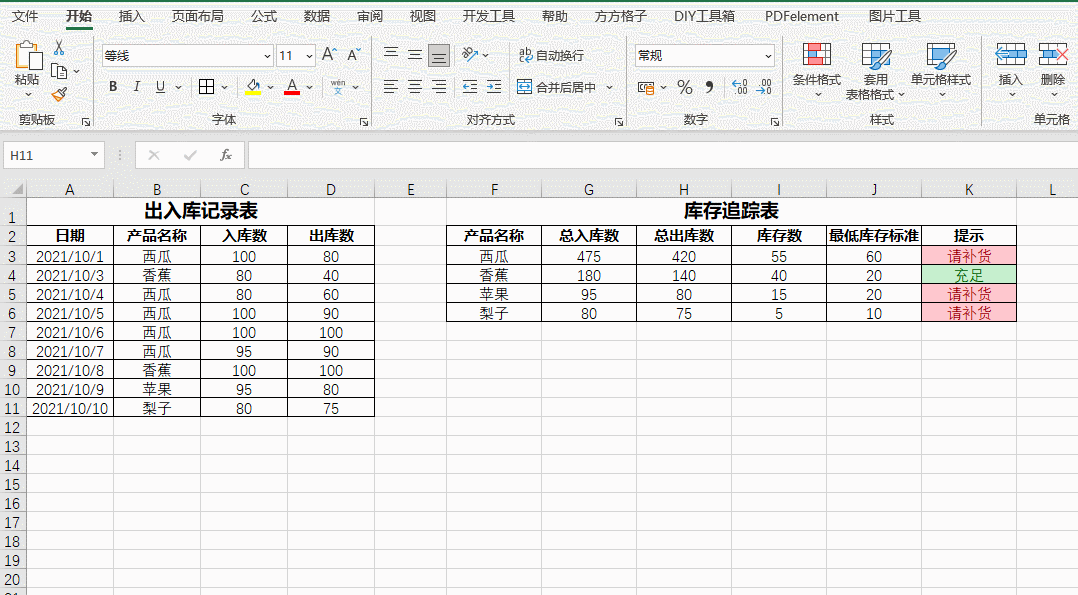## 制作出入库记录表## 制作库存追踪表## 函数解析

### 总入库数=IF(SUMIF($B:$B,F3,$C:$C)=0,"",SUMIF($B:$B,F3,$C:$C))

### 总出库数=IF(SUMIF($B:$B,F3,$D:$D)=0,"",SUMIF($B:$B,F3,$D:$D))

### 库存数=IFERROR(G3-H3,"")

### 提示=IF(J3="","",IF(I3<=J3,"请补货","充足"))## 最后的美化与修改

• 每次输完数据，还得额外加框线
• 表格公式需要每次单独填充END

本文作者：
文章标题：使用EXCEL制作简易的进销存表格
本文地址：https://www.cry33.com/archives/885.html
除非另有说明，本作品采用知识共享署名-非商业性使用-相同方式共享 4.0 国际许可协议
版权说明：若无注明，本文皆逍遥隐士原创，转载请保留文章出处。